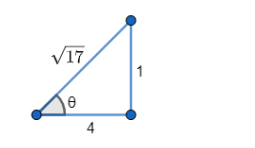# Prove that:

Question:

Prove that:

$\sin ^{-1} \frac{1}{\sqrt{17}}+\cos ^{-1} \frac{9}{\sqrt{85}}=\tan ^{-1} \frac{1}{2}$

Solution:

To Prove: $\sin ^{-1} \frac{1}{\sqrt{17}}+\cos ^{-1} \frac{9}{\sqrt{85}}=\tan ^{-1} \frac{1}{2}$

Formula Used: $\tan ^{-1} x+\tan ^{-1} y=\tan ^{-1}\left(\frac{x+y}{1-x y}\right)$ where $x y<1$

Proof:

$\mathrm{LHS}=\sin ^{-1} \frac{1}{\sqrt{17}}+\cos ^{-1} \frac{9}{\sqrt{85}} \ldots$ (1)

Let $\sin \theta=\frac{1}{\sqrt{17}}$Therefore $\theta=\sin ^{-1} \frac{1}{\sqrt{1} 7} \ldots$ (2)

From the figure, $\tan \theta=\frac{1}{4}$

$\Rightarrow \theta=\tan ^{-1} \frac{1}{4} \ldots$ (3)

From $(2)$ and $(3)$,

$\sin ^{-1} \frac{1}{\sqrt{17}}=\tan ^{-1} \frac{1}{4} \ldots$ (3)

Now, let $\cos \theta=\frac{9}{\sqrt{85}}$

Therefore $\theta=\cos ^{-1} \frac{9}{\sqrt{8} 5} \ldots$ (4)

From the figure, $\tan \theta=\frac{2}{9}$

$\Rightarrow \theta=\tan ^{-1} \frac{2}{9} \ldots$ (5)

From (4) and (5),

$\cos ^{-1} \frac{9}{\sqrt{85}}=\tan ^{-1} \frac{2}{9} \ldots$ (6)

Substituting (3) and (6) in (1), we get

$\mathrm{LHS}=\tan ^{-1} \frac{1}{4}+\tan ^{-1} \frac{2}{9}$

$=\tan ^{-1}\left(\frac{\frac{1}{4}+\frac{2}{9}}{1-\left(\frac{1}{4} \times \frac{2}{9}\right)}\right)$

$=\tan ^{-1}\left(\frac{9+8}{36-2}\right)$

$=\tan ^{-1} \frac{17}{34}$

$=\tan ^{-1} \frac{1}{2}$

$=\operatorname{RHS}$

Therefore, LHS = RHS

Hence proved.